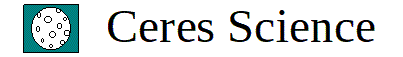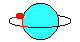AP Physics 1 Class Projects - Windows Applications - Version 2022 ©

Welcome to the AP Physics 1 Class Projects site. We designed several class projects of great value to physics teachers and students. Most projects are based on AP Physics problems that were given to the students in official AP Physics exams. We transformed the different problems into comprehensive learning activities that are not only motivating, but also of high academic value. For instance, the Bouncing Ball project, is a superb activity in mechanics that requires about 4 hours of intense work, and the application of concepts in projectile motion, conservation of momentum, and conservation of energy.

It is recommended that teachers give a different set of values for the students to use in each project. One good thing is that each project includes a tutorial, which explains in detail the work that needs to be done. In addition, each project also includes a Windows application that generates in seconds the answer keys of all the projects that were given to an entire class. Therefore, teachers will take just one minute or so to grade each project. We made these educational products very affordable to teachers. For just \$14.95 you can buy in Teachers Pay Teachers a class project that will give your students an unforgettable learning experience. You can choose what output with the answer keys is more convenient to you. In some projects the answer keys are shown on the computer screen. In other peojects, the output is a textfile, which you can edit and send to the printer.

The projects available are the following:

The Bouncing Ball

Rotation in a Vertical Plane

Rotation in a Horizontal Plane

Projectile Motion

Vectors

The Inclined Plane and the Spring

The Vertical Spring

The Pendulum

The Block on the Sphere

Gravitation

Links to switch to other parts of our web site are located at the bottom of this page.

The Bouncing Ball Class Project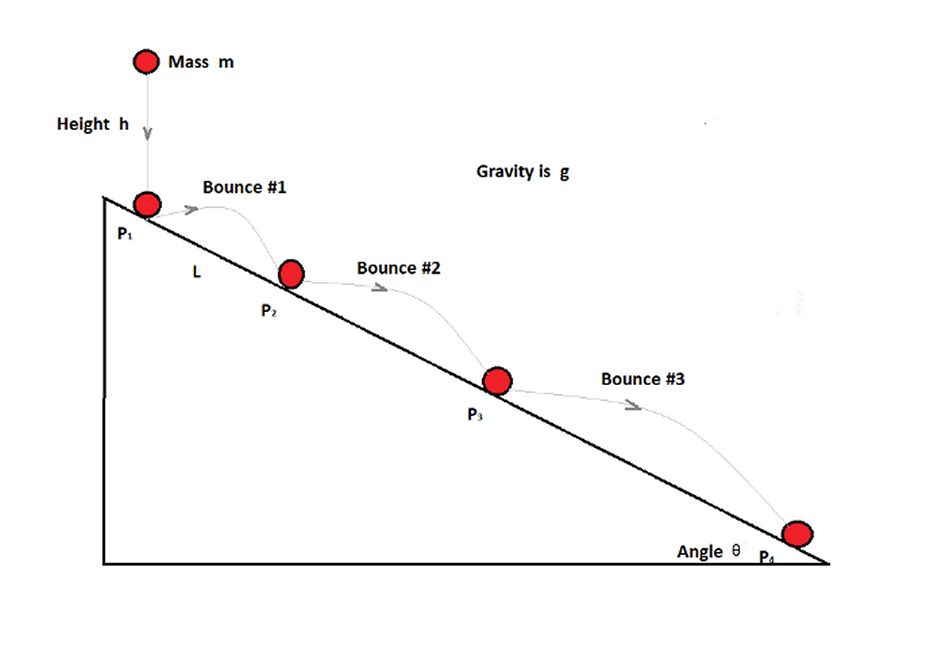A ball is released from a point above an inclined plane. The following information is known:

the mass m of the ball
the height
h above the inclined plane where the ball is released.
The angle of inclination
θ the plane makes with the horizontal.
The value of gravity
g

The ball is allowed to bounce 5 or more times down along the inclined plane. For each bounce, each student has to calculate several values. The first bounce is from point P1 to point P2, the second bounce from point P2 to point P3, the third bounce from P3 to point P4, and so on. All the bounces are perfectly elastic, thus, the ball experiences no loss of kinetic energy during each collision with the plane. And, of course, momentum is always conserved. Students will find 10 values in each bounce. For instance, during the second bounce between points P2 and P3, the students will calculate the following quantities (note that in this bounce the first point is P2 and the second point is P3):

The velocity of the ball at the first point - Variable VEL(P2)
The velocity of the ball at the second point - Variable VEL(P3)
The time the ball takes to travel between the two points - T
The distance traveled along the plane between the two points - Variable L
The horizontal velocity immediately after beginning the bounce - Variable Vx
The vertical velocity immediately after beginning the bounce - Variable Vy
The angle the ball makes with the vertical right before it bounces from the first point - Variable Beta
The angle the ball makes with the inclined plane right before or right after it bounces from the first point - Variable INC
The angle the ball makes with the horizontal immediately after it bounces from the first point - Variable ALPHA
Kinetic energy of the ball at the first point - Variable KE

The Bouncing Ball Class Project 1: The data for an entire class is entered in a text file in a few minutes, and the answer keys, one by one, are printed on the screen. A Vectors tutorial is included.
Link to The Bouncing Ball Class Project 1 in TpT         Cost: \$14.95

The Bouncing Ball Class Project 2: The data for an entire class is entered in a text file in a few minutes. All the answer keys are sent to a text file, which can be analyzed on the screen or printed on paper. A Vectors tutorial is included.
Link to The Bouncing Ball Class Project 2 in TpT         Cost: \$14.95

The Bouncing Ball Class Project 3: The data for one student is entered on the screen in a few seconds, and the answer key is printed on the screen right away. It includes a Vectors tutorial.
Link to The Bouncing Ball Class Project 3 in TpT         Cost: \$14.95

The Bouncing Ball Class Project 4: The data for one student is entered on the screen in a few seconds, and the answer key is sent to a text file. The content of the text file can be seen on the screen or sent to a printer. It includes a Vectors tutorial.
Link to The Bouncing Ball Class Project 4 in TpT         Cost: \$14.95

Rotation in a Vertical Plane Class Project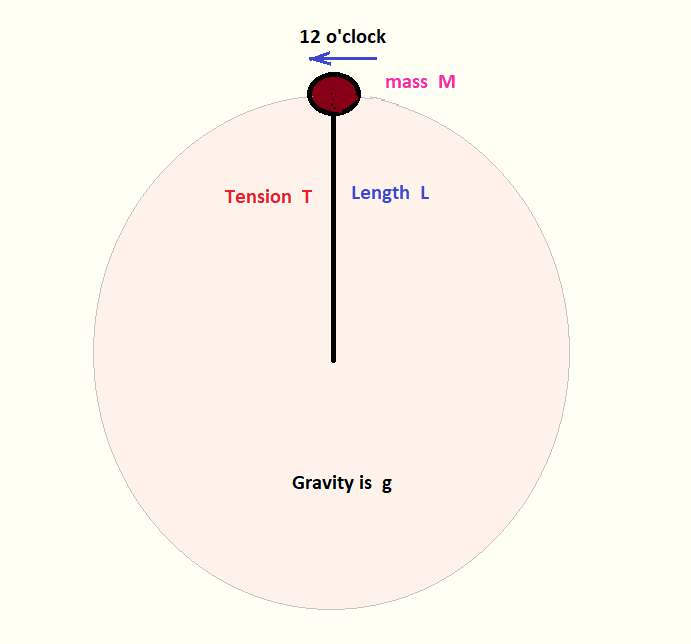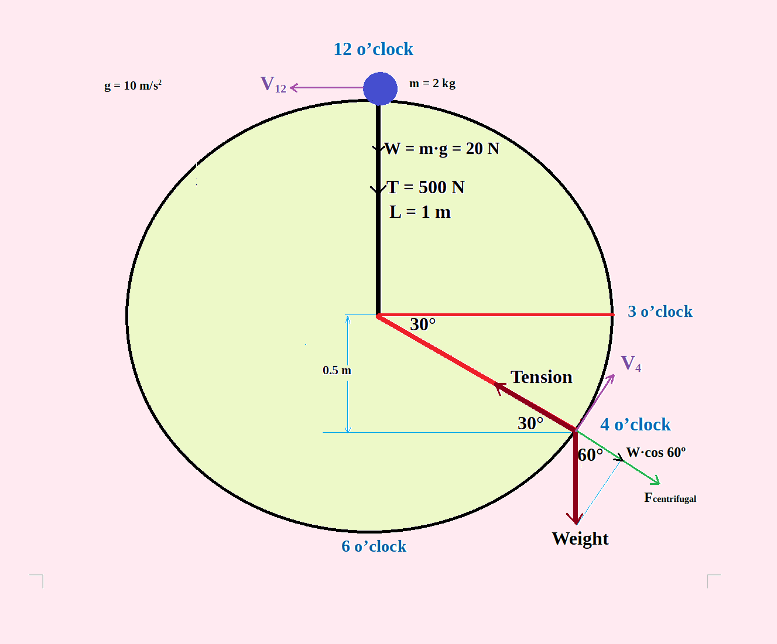A ball of mass M kg is attached at the end of a string, and it is rotating counterclockwise in a vertical plane. The following information is known:

the mass M of the rotating ball
the tension
T at the 12 o'clock position.
The length
L of the string.
The value of gravity
g

The purpose of this project is that for each hour of the clock, the students will find the following values:

Angular Displacement (measured from 12 o'clock counterclockwise)
Linear Displacement (measured from 12 o'clock counterclockwise)
Angular and Linear Velocity
Angular and Linear Acceleration
Tension in the string
Potential Energy (PE = 0 J 6 o'clock)
Kinetic Energy
Total Energy
Centripetal Force and Acceleration
Net Force and Direction of the Net Force
Angular Momentum and Moment of Inertia

Rotation in a Vertical Plane Project 1: The data for one student is entered on the screen in a few seconds, and the answer key is printed on the screen right away. The answer key includes the solution for every hour of the clock. This project incorporates a Rotation in a Vertical Plane tutorial.
Link to The Rotation in a Vertical Plane Class Project 1 in TpT         Cost: \$14.95

Rotation in a Vertical Plane Project 2: The data for one student is entered on the screen in a few seconds, and the answer key is printed on the screen and also in a text file. The answer key includes the solution for one hour of the clock. This project includes a Rotation in a Vertical Plane tutorial.
Link to The Rotation in a Vertical Plane Class Project 2 in TpT         Cost: \$14.95

Rotation in a Vertical Plane Project 3: The data for one student is entered on the screen in a few seconds, and the answer key is printed in a text file. The answer key includes the solution for every hour of the clock. This project includes a Rotation in a Vertical Plane tutorial.
Link to The Rotation in a Vertical Plane Class Project 3 in TpT         Cost: \$14.95

Rotation in a Vertical Plane Project 4: The data for one class is entered in a text file in a few minutes, and the answer keys are printed in individual text files. The answer keys include the solutions for every hour of the clock. This project includes a Rotation in a Vertical Plane tutorial.
Link to The Rotation in a Vertical Plane Class Project 4 in TpT         Cost: \$14.95

Rotation in a Horizontal Plane Class ProjectA block attached to a string is rotating on a horizontal surface. There is friction between the block and the surface. Eventually the block stops. The following information is known:

The mass of the block
Gravity
Length of the string
Initial angular velocity in rpm
Angular displacement (number of revolution to stop)

The following values will be determined:

Angular acceleration
Time to stop
Average force of friction
Total heat produced
Initial tension in the string

For every revolution the block travels until it stops, the computer also will calculate:

Linear Displacement
Angular Velocity
Linear Velocity
Angular Acceleration
Linear Acceleration
Tension in the String
Force of Friction
Heat Produced
Time Passed

Rotation in a Horizontal Plane Project: The data for one student is entered on the screen in a few seconds, and the answer key is printed on the screen right away.
Link to The Rotation in a Horizontal Plane Project in TpT         Cost: \$14.95

Projectile Motion Class ProjectA projectile is ejected at an angle above the horizontal and it will stay in flight for about 100 s. The following information is known:

the Initial Velocity Vo the projectile is thrown.
The angle of inclination
θ above the ground.
The value of gravity
g

The computer will calculate:

Time to reach the peak
Total Time the projectile is in flight
Total Range
Maximum Height

In addition, for each 5-second interval the computer will find:

Horizontal Velocity
Vertical Velocity
Speed
Range
Height
Angle of inclination
Linear acceleration

Besides the mathematical solution, the students also will do the graph Height vs. Time.

Projectile Motion Class Project 1: The data for one student is entered on the screen in a few seconds, and the answer key is printed on the screen right away. It includes a Projectile Motion tutorial.
Link to The Projectile Motion Class Project 1 in TpT         Cost: \$14.95

Projectile Motion Class Project 2: The data for one student is entered on the screen in a few seconds, and the answer key is printed in a text file right away. It includes a Projectile Motion tutorial.
Link to The Projectile Motion Class Project 2 in TpT         Cost: \$14.95

Projectile Motion Class Project 3: The data for one class is entered in a text file in a few minutes. The data entered includes an ID number for every student. The computer will use the ID numbers to generate different data at random for every student. The answer keys are printed in a text file. This project includes a Projectile Motion tutorial.
Link to The Projectile Motion Class Project 3 in TpT         Cost: \$14.95

Vectors Class Project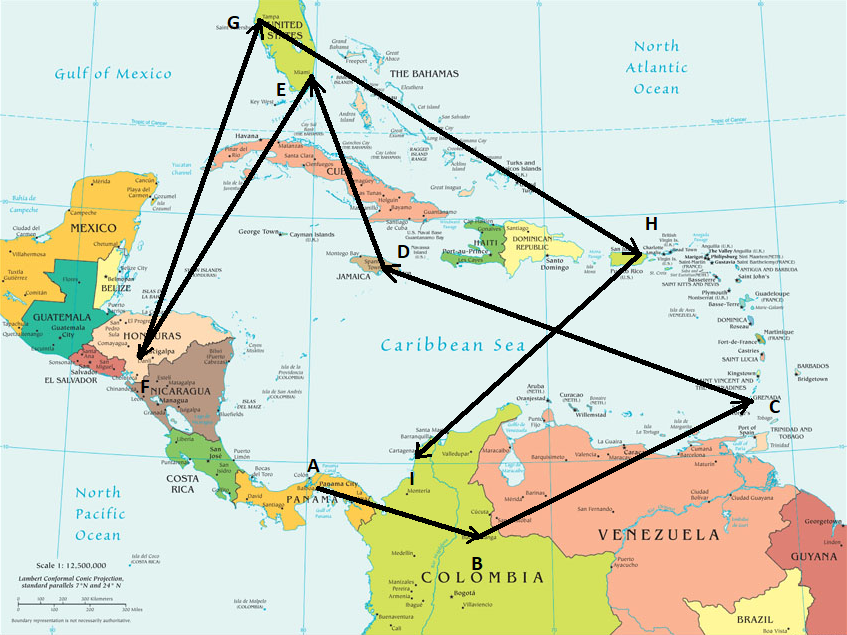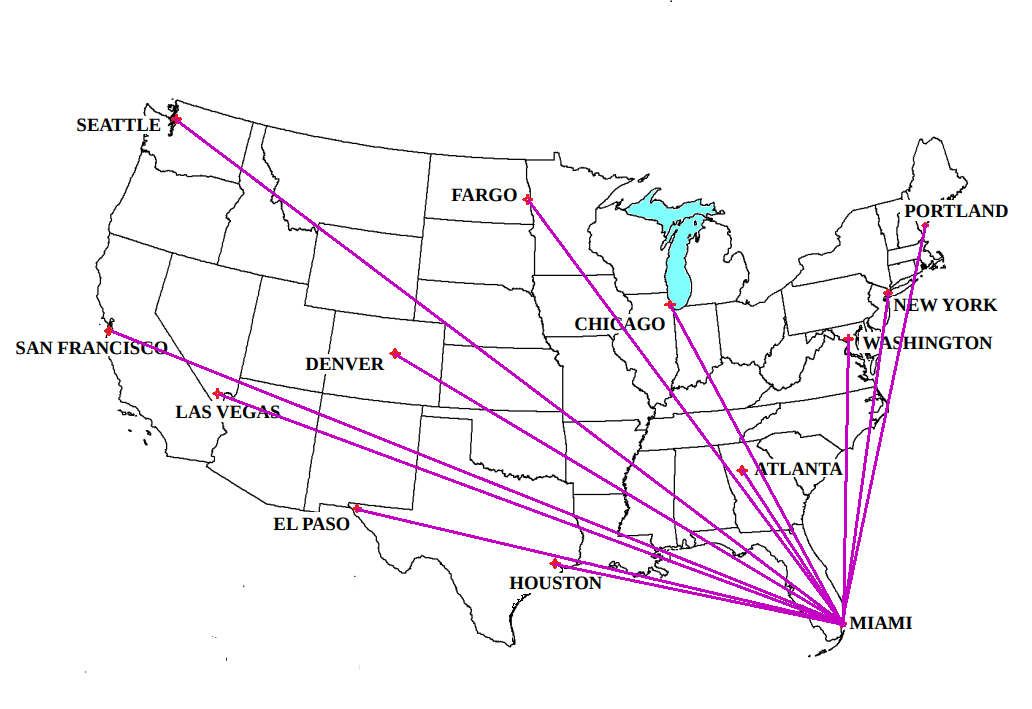The Vectors Class Project is about a hypothetical journey by airplane to many cities. First, the airplane travels from city A to city B, then from city B to city C, from city C to city D, and so on. For each pair of cities, for instance between cities B and C, the distance is known, as well as the direction of the second city in reference to the first. The main objective is for the students to calculate: (a) the distance between every city and city A, and (b) the direction of every city in reference to the first city A. In essence, students will practice the addition of 10 or more vectors. After they complete the mathematical solution, the students also will solve their vectors problems graphically. Everything is explained in a clear and well designed tutorial. In the table the students have to complete in the mathematical solution, the columns are the following:

Cities (from)
Cities (to)The velocity of the ball at the second point - Variable VEL(P3)
Distance
Direction
Horizontal Projection
Vertical Projection
Summation of Horizontal Projections
Summation of Horizontal Projections
Resultant (Distance)
Resultant (Direction)

Vectors Class Project 1: The data (distance and direction) for one student is entered on the screen in a few seconds for each vector, and each time the Resultant is printed on the screen. The limit is 25 vectors. The project includes a well designed tutorial.
Link to Vectors Class Project 1 in TpT         Cost: \$14.95

Vectors Class Project 2: The data for one class is entered in a text file in a few minutes. The data entered includes an ID number for every student. The computer will use the ID numbers to generate different data for every student following specific rules. The answer keys are printed on the screen one by one. This project includes a Vectors Tutorial.
Link to Vectors Class Project 2 in TpT         Cost: \$14.95

Vectors Class Project 3: The data for one student is entered on the screen in a few seconds. The data entered includes an ID number. The computer will use the ID numbers to generate different data for the student following specific rules. The answer key sent to a text file. This project includes a Vectors Tutorial.
Link to Vectors Class Project 3 in TpT         Cost: \$14.95

Vectors Class Project 4: The data for one class is entered in a text file in a few minutes. The data entered includes an ID number for every student. The computer will use the ID numbers to generate different data for every student following specific rules. The answer keys are printed in a text file. This project includes a Vectors Tutorial.
Link to Vectors Class Project 4 in TpT         Cost: \$14.95

Vectors Class Project 5: The data for one class is entered in a text file in a few minutes. The data entered include the names of the students, and the distances and directions between consecutive cities in the journey. The answer keys are printed on the screen one by one. This project includes a Vectors Tutorial.
Link to Vectors Class Project 5 in TpT         Cost: \$14.95

Vectors Class Project 6: The data for one class is entered in a text file in a few minutes. The data entered includes the names of the students, and the distance and direction between consecutive cities in the journey. The answer keys are printed in a text file. This project includes a Vectors Tutorial.
Link to Vectors Class Project 6 in TpT         Cost: \$14.95

The Inclined Plane and the Spring Class Project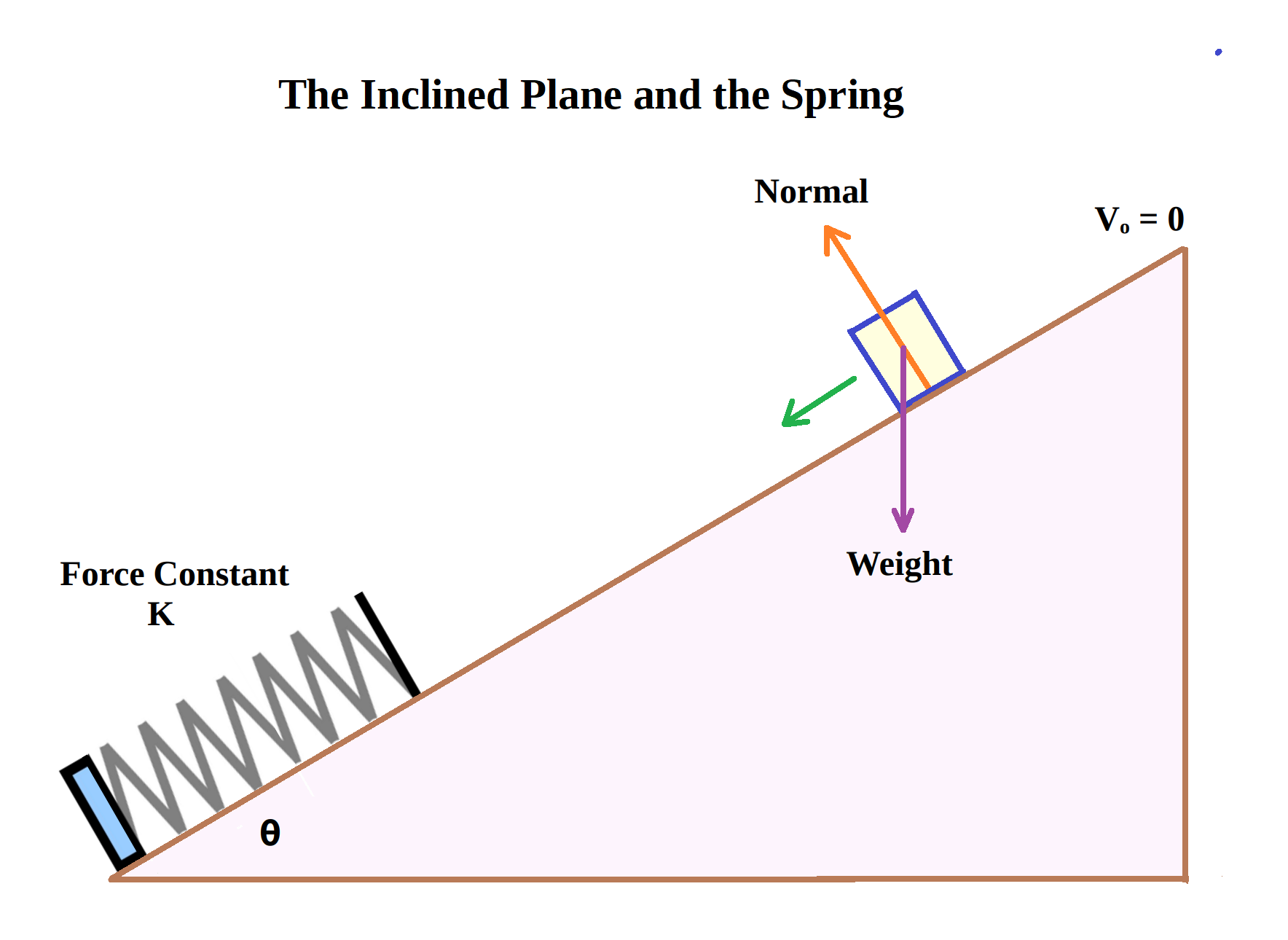A spring is placed at the bottom of an inclined plane. Starting from rest, a block of mass M is allowed to slide down the frictionless plane. After traveling the distance d down along the plane, the block starts compressing the spring. One good question to ask is: where does the block have the greatest speed from the moment it starts sliding to the moment it is stopped by the spring?

The following values need to be entered:

the mass M of the block.
the force constant K of the spring.
The initial separation
d between the block and the spring.
The angle of inclination
θ of the plane.
The value of gravity
g.

The computer will estimate these values:

The acceleration of the block
The velocity at the instant the block strikes the spring
The maximum length L the spring is compressed by the block

From the moment the moving block starts compressing the spring, for every centimeter the spring is compressed, and until the block stops, the computer will calculate the following quantities:

Speed of the block
Kinetic Energy of the block
Net Force acting on the block
Acceleration of the block
Potential Energy stored in the spring

All the results will be printed on the screen.

The Inclined Plane and the Spring Project: The data for one student is entered on the screen in a few seconds, and the answer key is printed on the screen right away. It includes a tutorial that explains step by step the solution of this problem.
Link to The Inclined Plane and the Spring Project in TpT         Cost: \$14.95

The Vertical Spring Class Project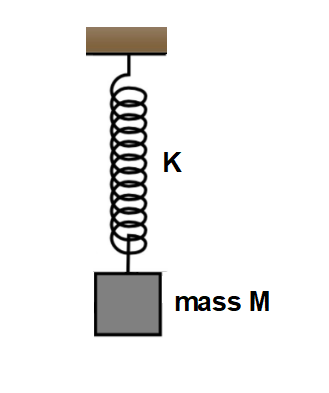A light vertical spring has an original unstretched length Lo. A block of mass M is carefully attached to its lower end, and the block comes to rest when the new length of the spring is L. Finally, the block is pulled down the distance X, and then it is released, causing the block to oscillate up and down from the equilibrium line.

The application will ask to enter the following data:

the mass M of the block.
the initial length of the spring
Lo.
the new length of the spring
L.
x the spring is stretched.
The value of gravity
g.

The following quantities will be calculated:

the force constant K of the spring.
the frequency
f.
the period
T.
the maximum speed of the block
V.
the maximum acceleration
a of the block.

For convenience, the student will assume that the block has zero Potential Energy PE when it is located at the lowest point of each oscillation. Starting at the lowest point, for every centimeter the block moves, the following values will be calculated:

Potential Energy of the block
Kinetic Energy of the block
Potential Energy stored in the spring
Velocity of the block
Acceleration of the block

It is recommended that teachers give a different set of values to each student. To practice graphing, the students will do the graph Displacement ( -x to +x ) vs. PE (block), KE (block), PE (spring), and TE.

The Vertical Spring Project 1: The data for one student is entered on the screen in a few seconds, and the answer key is printed on the screen and also sent to a textfile right away. It includes a tutorial that explains step by step the solution of this problem.
Link to The Vertical Spring Project 1 in TpT         Cost: \$14.95

The Vertical Spring Project 2: The data for one student is entered on the screen in a few seconds, and the answer key is printed on the screen right away. It includes a tutorial that explains step by step the solution of this problem.
Link to The Vertical Spring Project 2 in TpT         Cost: \$14.95

The Pendulum Class Project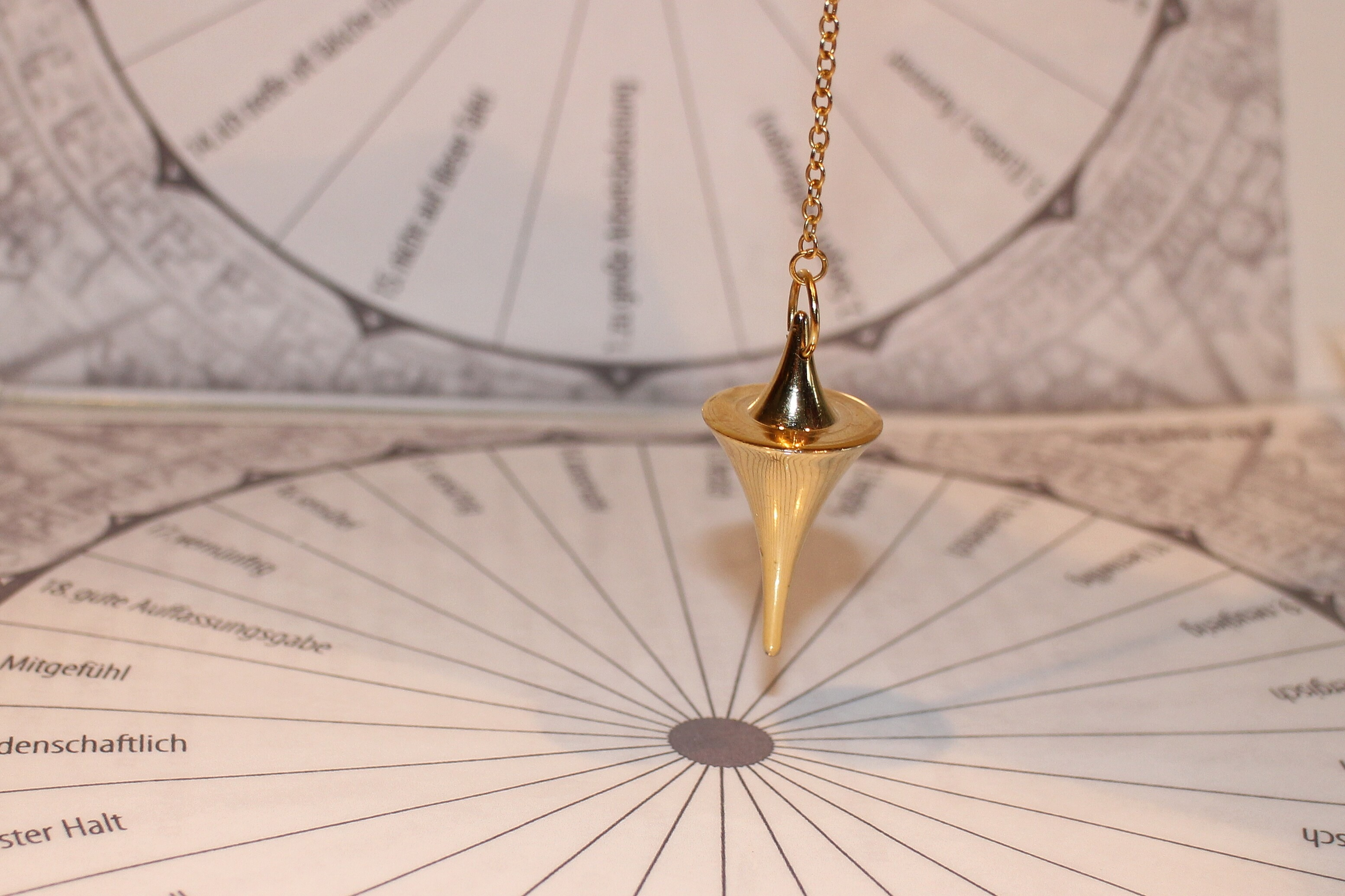The bob of a pendulum is released and the pendulum keeps swinging back and forth.

The application will ask to enter the following values:

the mass m of the bob.
the length
L of the string.
the angle
θ with the vertical the pensulum is released.
The value of gravity
g.

The computer will find:

Period and Frequency
The Maximum Velocity
Maximum Kinetic Energy

For every degree the pendulum moves, the following values are calculated:

Velocity
Kinetic Energy KE
Potential Energy PE
Total Energy TE
Centrifugal Force
Tension in the string
Net Force
Linear Acceleration
Angular Acceleration

All the results will be printed on the screen.

The Pendulum Project: The data for one student is entered on the screen in a few seconds, and the answer key is printed on the screen right away. It includes a tutorial that explains step by step the solution of this problem.
Link to The Pendulum Project in TpT         Cost: \$14.95

The Block on the Sphere Class Project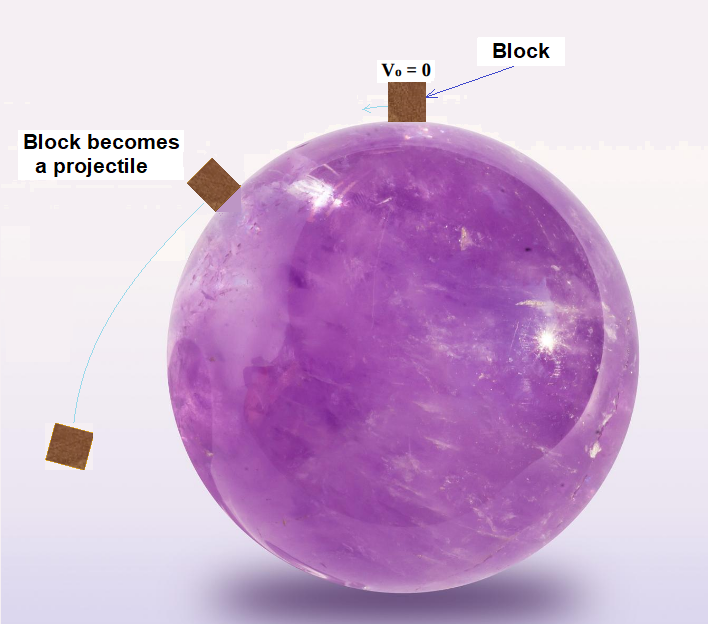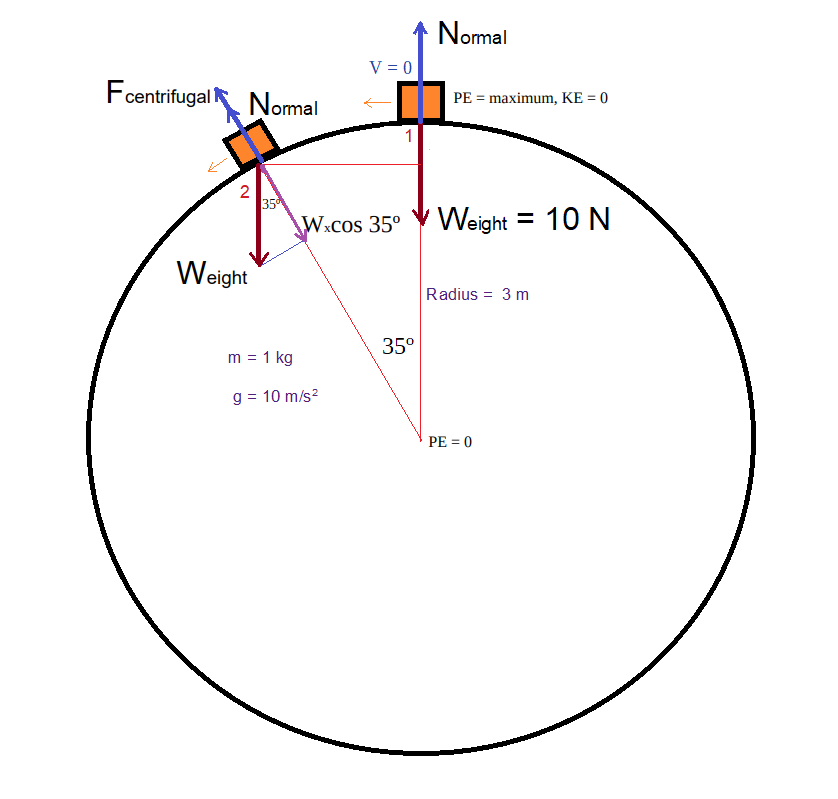A small block is placed on top of a large sphere. The block is then touched very softly, causing it to slide, starting from rest, on the frictionless surface of the sphere. Eventually, the block loses contact with the surface of the sphere and becomes a projectile.

The application will ask to enter the following data:

the mass m of the block.
R of the sphere.
The value of gravity
g.

From the moment the block is released on top of the sphere, to the moment the block becomes a projectile after traveling an arc in degrees that needs to be estimated, for every 5-degree segment the block slides, the computer will calculate the following quantities:

the Potential Energy PE (PE of the block is zero at the center of the sphere).
the Kinetic Energy
KE of the block.
the Total Energy
TE.
the Velocity
V of the block.
the linear acceleration
a.
the Centrifugal Force
F.
the Normal Force
N.

The Block on the Sphere Project 1: The data for one student is entered on the screen in a few seconds, and the answer key is printed on the screen right away. It includes a tutorial that explains step by step the solution of this problem.
Link to The Block on the Sphere Project 1 in TpT         Cost: \$14.95

The Block on the Sphere Project 2: The data for one student is entered on the screen in a few seconds, and the answer key is printed on the screen and also sent to a text file right away. It includes a tutorial that explains step by step the solution of this problem.
Link to The Block on the Sphere Project 2 in TpT         Cost: \$14.95

Gravitation Class ProjectThe Gravitation projects described below generate a significant amount of astronomical data for the planets Earth, Mars, Jupiter, and Saturn. Each project includes a comprehensive 17-page tutorial in Gravitation, which explains in detail every concept, in addition to 12 numerical exercises. The following information about the planet is available and used by the corresponding application:

Sidereal Period of Revolution
Period of Rotation around its own axis
Mass

Initially, each application calculates the following:

Strength of gravity on the surface
Average density
Altitude of an isosynchronous satellite
Average orbital velocity
Period of a satellite at an altitude of 1,000 km
Escape velocity

The main feature of each application is that for any altitude that is entered (from 0 km to 1 million km), the computer will estimate the following values:

Strength of Gravity
Orbital Velocity
Orbital Period
Centripetal acceleration

All the results are printed on the screen

Gravitation Project 1 - Planet Earth
Link to The Gravitation Project 1 - Planet Earth in TpT         Cost: \$14.95

Gravitation Project 2 - Planet Mars
Link to The Gravitation Project 2 - Planet Mars in TpT         Cost: \$14.95

Gravitation Project 3 - Planet Jupiter
Link to The Gravitation Project 3 - Planet Jupiter in TpT         Cost: \$14.95

Gravitation Project 4 - Planet Saturn
Link to The Gravitation Project 4 - Planet Saturn in TpT         Cost: \$14.95

Home Page      Physics Worksheets      Chemistry      Biology      Physical Science      Links to Ceres Science in TpT      AP Physics 1 Projects
Earth & Space Science      Physics Test Generators       Physics Video Exams      Anatomy         Balsa Wood Bridges      Electric Motors
Computer Science Worksheets       Health Science        Zoology        View & Play Activities       Free Materials       Boards in Pinterest
BASIC Language       Physics Applications        Chemistry Applications        Physics Lessons       All Science Products       To Facebook